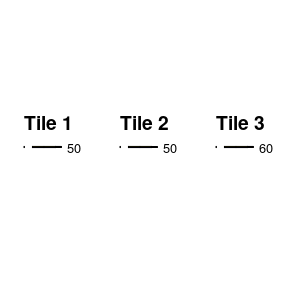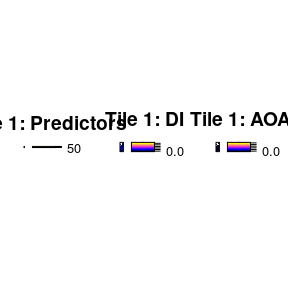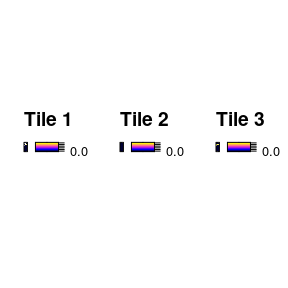# 3. AOA in Parallel

#### 2022-08-24

Estimating the Area of Applicability (AOA) can be computationally intensive, depending on the amount of training data used for a model as well as the amount of new data the AOA has to be computed. This vignette goes over the possibility to (partly) compute the AOA in parallel. We will use the same data setup as the vignette “Area of applicability of spatial prediction models”. Please have a look there for a general introduction to the AOA and the details about the example data generation.

# Generate Example Data

library(CAST)
library(virtualspecies)
library(caret)
library(raster)
library(sp)
library(sf)
library(viridis)
library(latticeExtra)
library(gridExtra)
predictors <- stack(system.file("extdata","bioclim.grd",package="CAST"))
response <- generateSpFromPCA(predictors,
means = c(3,1),sds = c(2,2), plot=F)$suitab.raster mask <- predictors[] values(mask)[!is.na(values(mask))] <- 1 mask <- rasterToPolygons(mask) # Generate Clustered Training Samples csample <- function(x,n,nclusters,maxdist,seed){ set.seed(seed) cpoints <- sp::spsample(x, n = nclusters, type="random") result <- cpoints result$clstrID <- 1:length(cpoints)
for (i in 1:length(cpoints)){
ext <- rgeos::gBuffer(cpoints[i,], width = maxdist)
newsamples <- sp::spsample(ext, n = (n-nclusters)/nclusters,
type="random")
newsamples$clstrID <- rep(i,length(newsamples)) result <- rbind(result,newsamples) } result$ID <- 1:nrow(result)
return(result)
}

trainDat <- extract(predictors,samplepoints,df=TRUE)
trainDat$response <- extract (response,samplepoints) trainDat <- merge(trainDat,samplepoints,by.x="ID",by.y="ID") trainDat <- trainDat[complete.cases(trainDat),] set.seed(10) model_random <- train(trainDat[,names(predictors)], trainDat$response,
method="rf",
importance=TRUE,
trControl = trainControl(method="cv"))
prediction_random <- raster::predict(predictors,model_random)

# Parallel AOA 1: Providing a Cluster

The simplest methods to compute the AOA in parallel is by providing a cluster object in the function call. This way the distance calculation between training data and new data will run on multiple cores (utilizing parallel::parApply). This is recommended if the training set is relatively small but new locations for which the AOA should be computed are abundant.

library(doParallel)
library(parallel)
cl <- makeCluster(4)
registerDoParallel(cl)
AOA <- aoa(studyArea,model,cl=cl)

# Parallel AOA 2: Divide and Conquer

For even better performances, it is recommended to compute the AOA in two steps. First, the DI of training data and the resulting DI threshold is computed from the model or training data with the function trainDI. The result from trainDI is usually the first step of the aoa function, however it can be skipped by providing the trainDI object in the function call. This makes it possible to compute the AOA on multiple raster tiles at once (e.g. on different cores). This is especially useful for very large prediction areas, e.g. in global mapping.

model_random_trainDI = trainDI(model_random)
print(model_random_trainDI)
## DI of 74 observation
## Predictors: bio2 bio5 bio10 bio13 bio14 bio19
##
## AOA Threshold: 0.09237258
saveRDS(model_random_trainDI, "path/to/file")

If you have a large raster, you divide it into multiple smaller tiles and apply the trainDI object afterwards to each tile.

r1 = crop(predictors, c(0,7,42.33333,54.83333))
r2 = crop(predictors, c(7,14,42.33333,54.83333))
r3 = crop(predictors, c(14,21,42.33333,54.83333))

grid.arrange(spplot(r1[], main = "Tile 1"),
spplot(r2[], main = "Tile 2"),
spplot(r3[], main = "Tile 3"), ncol = 3)Use the trainDI argument in the aoa function to specify, that you want to use a previously computed trainDI object.

aoa_r1 = aoa(newdata = r1, trainDI = model_random_trainDI)

grid.arrange(spplot(r1[], main = "Tile 1: Predictors"),
spplot(aoa_r1$DI, main = "Tile 1: DI"), spplot(aoa_r1$AOA, main = "Tile 1: AOA"), ncol = 3)You can now run the aoa function in parallel on the different tiles! Of course you can use for favorite parallel backend for this task, here we use mclapply from the parallel package.

library(parallel)

tiles_aoa = mclapply(list(r1, r2, r3), function(tile){
aoa(newdata = tile, trainDI = model_random_trainDI)

}, mc.cores = 3)
grid.arrange(spplot(tiles_aoa[]$AOA, main = "Tile 1"), spplot(tiles_aoa[]$AOA, main = "Tile 2"),
spplot(tiles_aoa[]\$AOA, main = "Tile 3"), ncol = 3)For larger tasks it might be useful to save the tiles to you hard-drive and load them one by one to avoid filling up your RAM.

# Simple Example Code for raster tiles on the hard drive

tiles = list.files("path/to/tiles", full.names = TRUE)

tiles_aoa = mclapply(tiles, function(tile){
current = raster::stack(tile)
aoa(newdata = current, trainDI = model_random_trainDI)

}, mc.cores = 3)

# Final Remarks

It should be possible to combine both parallelization methods! For example on a High Performance Cluster, multiple raster tiles can be handled by different nodes and then, each node computes the distance between prediction and training data on multiple cores.